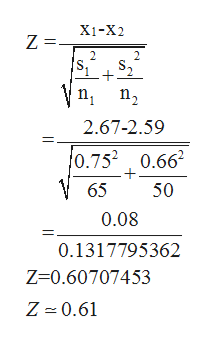# A sample of 65 observations is selected from one population with a population standard deviation of 0.75. The sample mean is 2.67. A sample of 50 observations is selected from a second population with a population standard deviation of 0.66. The sample mean is 2.59. Conduct the following test of hypothesis using the 0.08 significance level.H0 : μ1 ≤ μ2H1 : μ1 > μ2Compute the value of the test statistic. (Round your answer to 2 decimal places.) 2. What is the p-value? (Round your answer to 4 decimal places.)

Question
51 views

A sample of 65 observations is selected from one population with a population standard deviation of 0.75. The sample mean is 2.67. A sample of 50 observations is selected from a second population with a population standard deviation of 0.66. The sample mean is 2.59. Conduct the following test of hypothesis using the 0.08 significance level.

H0 : μ1 ≤ μ2

H1 : μ1 > μ2

1. Compute the value of the test statistic. (Round your answer to 2 decimal places.)

2. What is the p-value? (Round your answer to 4 decimal places.)

check_circle

Step 1

From the given information, level of significance = 0.08.

and

For a sample of 65,

n = 65

Mean =2.67 and standard deviation = 0.75.

For a sample of 50,

n = 50

Mean = 2.59 and Standard deviation = 0.66

Step 2

(1)

Formula for test statistic is,help_outlineImage TranscriptioncloseXi-X2 Z= 2. n n2 2.67-2.59 0.752 0.66 65 50 0.08 0.1317795362 Z-0.60707453 Z 0.61 fullscreen
Step 3

Therefore, test statistic is...

### Want to see the full answer?

See Solution

#### Want to see this answer and more?

Solutions are written by subject experts who are available 24/7. Questions are typically answered within 1 hour.*

See Solution
*Response times may vary by subject and question.
Tagged in

### Statistics